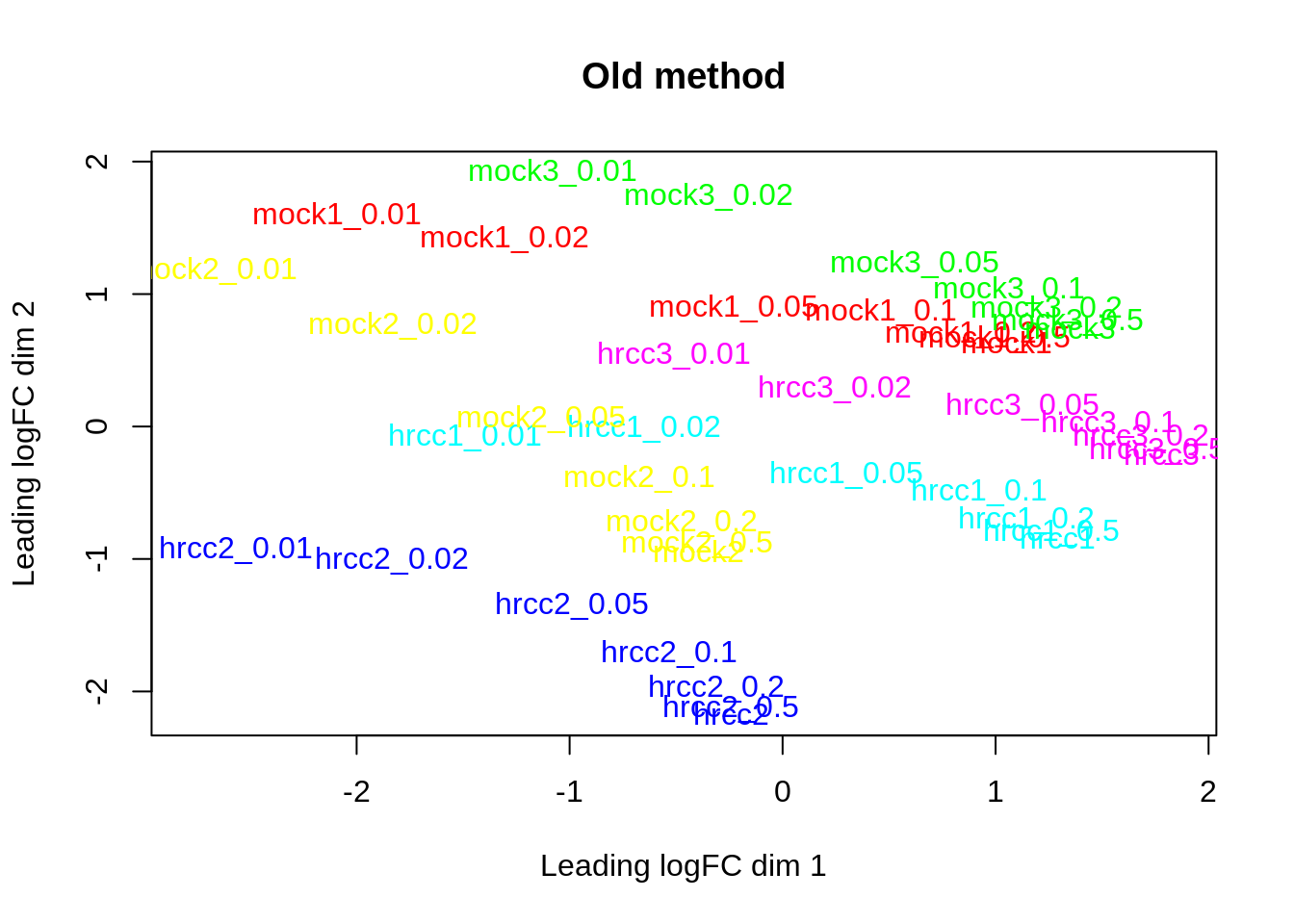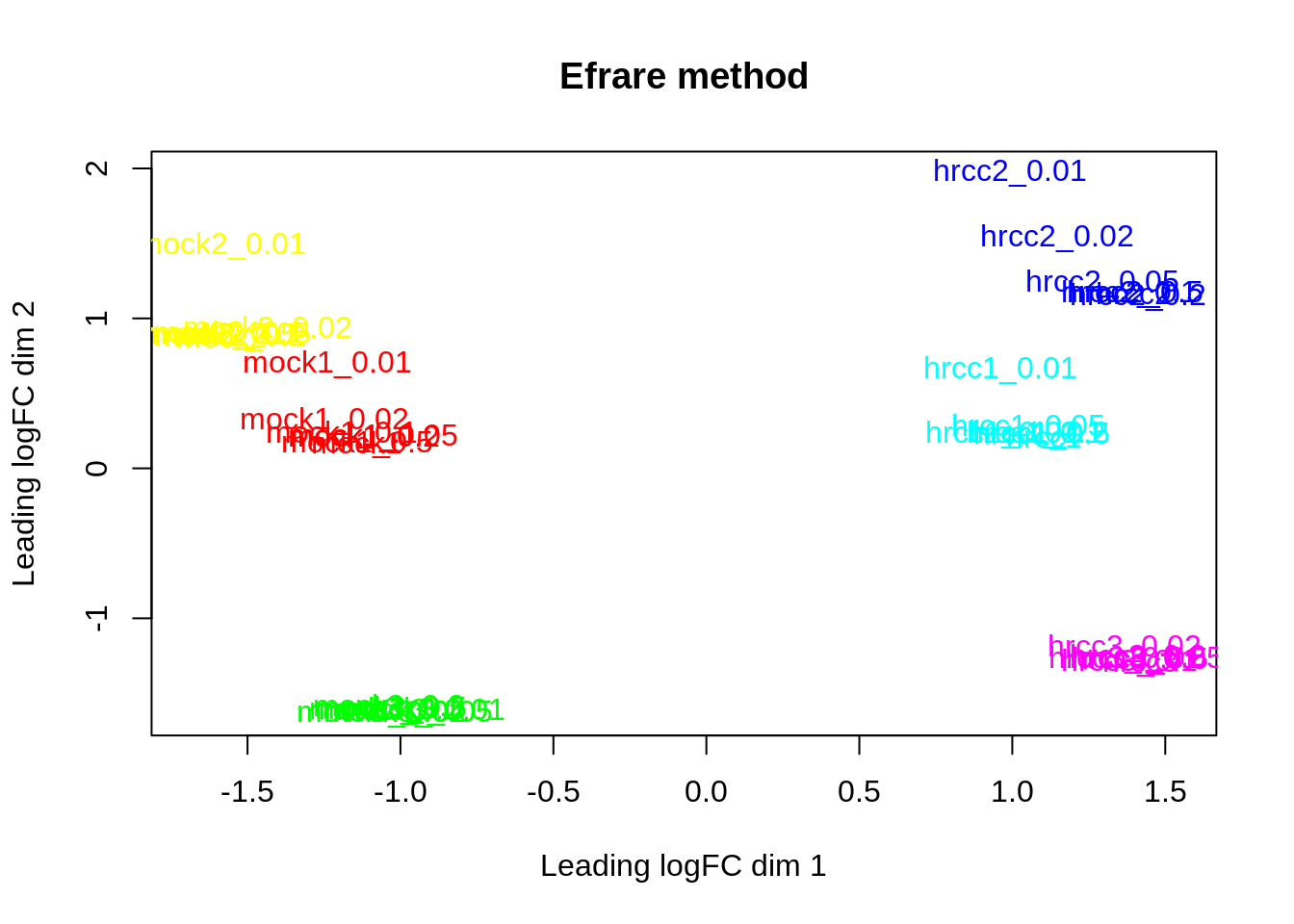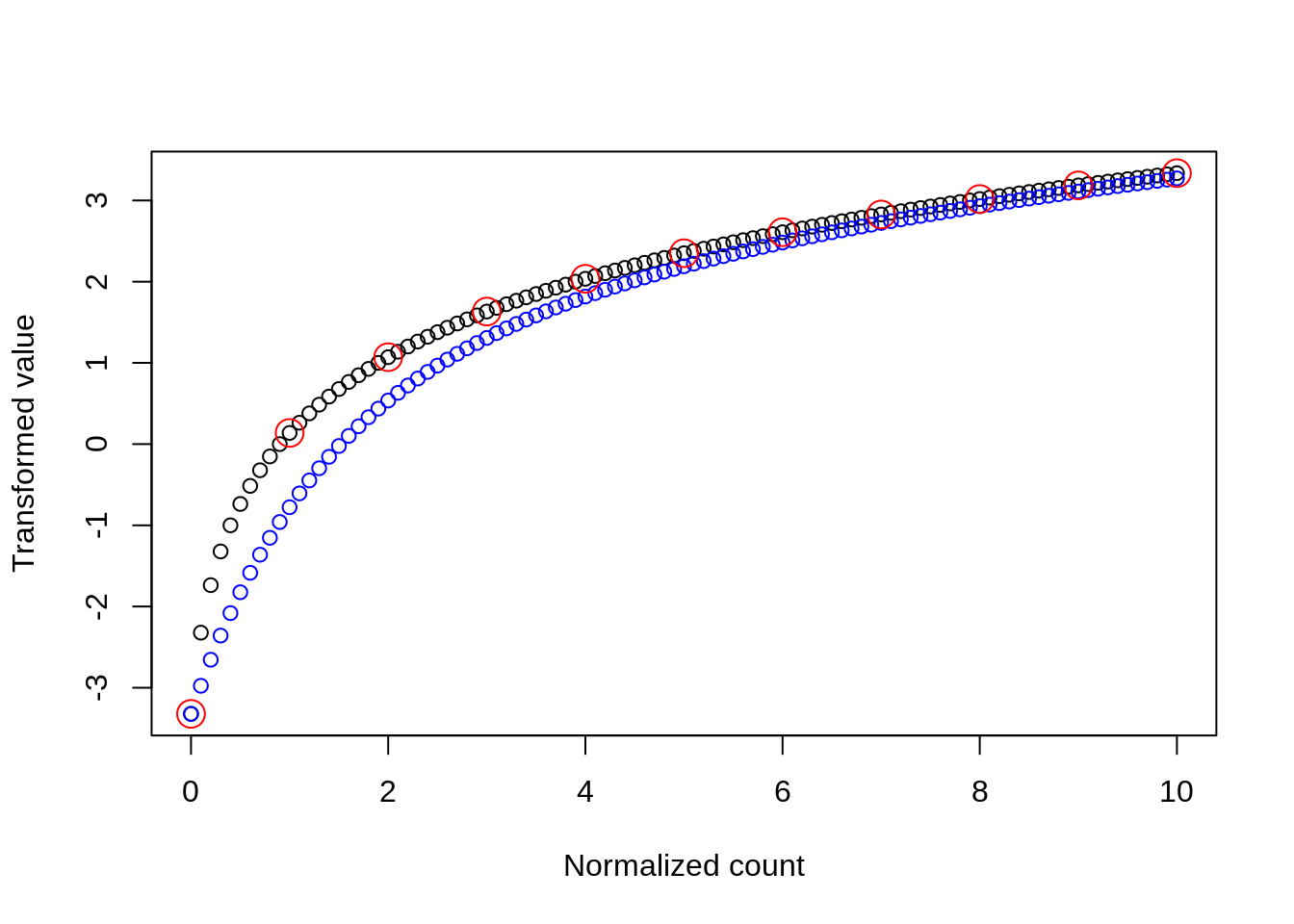This is an improved way to calculate log2(normalized_count+pseudocount) that removes a bias between samples due to differing library sizes (normalization amount). The idea is to use the expectation from rarefying to the smallest library size and then applying the log transformation.

It’s a small improvement, but we use this a lot in RNA-Seq and scRNA-Seq.

Rarefication is performed using the binomial distribution to sub-sample. (Hypergeometric sampling could be used but this requires a strict idea of library sizes. Using the binomial, we just need the subsampling fraction, which could be derived from a TMM-adjusted library size, or a particular normalizing gene, etc.)

The concept of rarefication uses random sampling, but actually we can calculate the expectation exactly. My code does this up to a certain count (max_exact argument), then approximates the remainder. Use max_exact=-1 for the old, uncorrected method.

# Implementation

``````log2plus <- function(add) function(count) log2(count+add)

#Expectation of f(rbinom(length(count),count,fraction))
#Calculate by summing dbinom(i,count,fraction)*f(i)
#Exact summation up to max_exact, then the remaining mean is transformed
efrare_vec <- function(counts, fraction, f=log2plus(1), max_exact=10) {
result <- numeric(length(counts))
mean_remainder <- counts*fraction
p_remainder <- rep(1,length(counts))
i <- 0
while(i <= max_exact) {

i <- i+1
}

# Could have used counts > max_exact,
# but numerical accuracy issues also arise as p->0
result
}

#Apply E(f(rarefied)) method to a matrix
efrare <- function(counts, fractions, f=log2plus(1), max_exact=10) {
result <- vector("list",ncol(counts))
for(i in seq_len(ncol(counts))) {
result[[i]] <- efrare_vec(counts[,i], fractions[i], f=f, max_exact=max_exact)
}
result <- do.call(cbind, result)
colnames(result) <- colnames(counts)
rownames(result) <- rownames(counts)
result
}``````

# Test with NBPSeq::arab dataset, small pseudocount

I rarefiy an RNA-Seq count matrix by various fractions (0.01,0.02,0.05,0.1,0.2,0.5,1), then apply the transformation.

The usual convention is to choose a pseudocount of 1 based on the mean library size.

To simulate this, I use a pseudocount of min(lib_sizes)/mean(lib_sizes) after normalizing to min(lib_sizes).

``````library(NBPSeq)
library(limma)
set.seed(1234)

rarefy <- function(counts, fraction) {
for(i in seq_len(ncol(counts)))
counts[,i] <- rbinom(nrow(counts), counts[,i], fraction)
colnames(counts) <- paste0(colnames(counts),"_",fraction)
counts
}

# arab dataset from NBPSeq, and various downsamplings of it
data(arab)
arab_down <- cbind(
rarefy(arab, 0.01),
rarefy(arab, 0.02),
rarefy(arab, 0.05),
rarefy(arab, 0.1),
rarefy(arab, 0.2),
rarefy(arab, 0.5),
arab)

lib_sizes <- colSums(arab_down)
fractions <- min(lib_sizes) / lib_sizes

# Pseudo-count of 0.01 in smallest library
# The idea is to simulate the effect of a pseudo-count of one in
# a small library if normalization was to the average library size.
pseudocount <- min(lib_sizes)/mean(lib_sizes)
pseudocount``````
``##  0.0206204``
``````f <- log2plus(pseudocount)

conventional <- f(sweep(arab_down, 2, fractions, "*"))
# or conventional <- efrare(arab_down, fractions, f=f, max_exact=-1)
plotMDS(conventional, col=rep(rainbow(6),7), main="Old method")````````````efrared <- efrare(arab_down, fractions, f=f, max_exact=10)
plotMDS(efrared, col=rep(rainbow(6),7), main="Efrare method")``````# What efrare does

Consider a rarefication to 10% then a pseudocount of 0.1.

``````f <- log2plus(0.1)

i <- 0:100
conventional <- efrare_vec(i, 0.1, f, max_exact=-1)
efrared <- efrare_vec(i, 0.1, f)
unrared <- f(i)

plot(i/10, conventional, xlab="Normalized count", ylab="Transformed value")
points(i/10, efrared, col="blue")
points(i, unrared, col="red", cex=2)``````• Red points: unrarefied transformation log2(count+0.1)
• Black points: log2(count*0.1+0.1) for a library 10 times larger than red
• Blue points: E(log2(rarefied count+0.1)) for a library 10 times larger than red

You can see the transformation is different based on the rarefication amount.

# Using a pseudocount of 1 with the smallest library size is also fine

The usual convention is to apply the pseudocount after normalizing to the mean library size. If we instead normalize to the smallest library size, a pseudocount of 1 is fine.

(However this may be inefficient if only one or two samples have a small library size. Hence the usual convention as above, letting these small samples be noisy in order to extract the most information from the rest of the samples.)

``````f <- log2plus(1)

conventional <- efrare(arab_down, fractions, f=f, max_exact=-1)
plotMDS(conventional, col=rep(rainbow(6),7), main="Old method, pseudocount 1 in smallest lib")``````